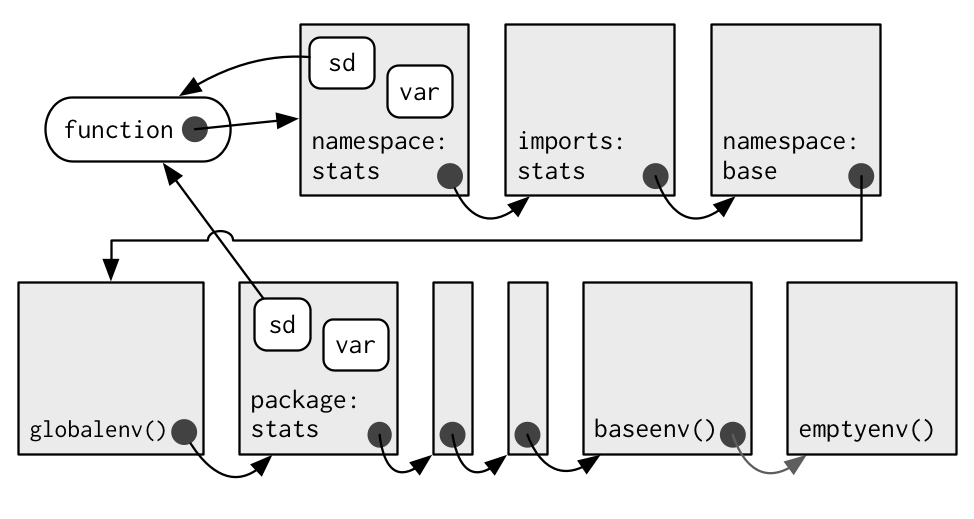# Some thoughts on tidyveal and environments in R

The tidyeval framework is a rather new, and in parts complementary, framework to dealing with non-standarde evaluation (NSE) in R. In short, NSE is about capturing some R-code, witholding execution, maybe editing the code, and finally execuing it later and/or somewhere else.

This post borrows heavily by Edwin Thon’s great post, and this post by the same author.

The typical base R culprits are `eval()`, and `quote()` or `substitute()`, respectively.

``````library(tidyverse)  # load tidyeval too
library(rlang)  # more on tidyeval
library(pryr)  # working with environments
``````
``````##
## Attaching package: 'pryr'
``````
``````## The following object is masked from 'package:rlang':
##
##     bytes
``````
``````## The following objects are masked from 'package:purrr':
##
##     compose, partial
``````

# What’s the difference between base nse and tidyeval nse?

And why should I bother?

Let’s look at some border case that show the difference quite strikingly.

Consider this base nse code:

``````base_NSE_fun <- function(some_arg) {
some_var <- 10
quote(some_var + some_arg)
}
base_NSE_fun(4) %>% eval()
``````
``````## Error in eval(.): object 'some_var' not found
``````

It does not work. `eval()` has no idea about the environment in which `some_var` is defined. (And in addition, `quote()` will take its arguments too literally, but we spare that for later.)

We might had expected the code above to work, however. That’s where tidyeval comes into play:

See here:

``````tidy_eval_fun <- function(some_arg) {
some_var <- 10
quo(some_var + some_arg)
}
tidy_eval_fun(4) %>% rlang::eval_tidy()
``````
``````##  14
``````

Does work. With tidyeval, the environment of the variables are memorized. With base R, the environment is forgotton.

# Looking at the chain of environments

What’s the enclosing environment of `eval()`? We can get hold of that environment using the function `environment()`:

``````environment(eval)
``````
``````## <environment: namespace:base>
``````

What’s that for a strange environment? Well, that’s the NAMESPACE of package `base`, the exported objects of that package. A namespace is useful so the searchpath will not be cluttered by too many objects. Using a namespace we can define which objects should be made (easily) accessible to the users.

But in this environment, for sure, we will not have put some variable. How comes that this code does wor:

``````vanilla_x <- 1
eval(vanilla_x)
``````
``````##  1
``````

What’s the environment of `vanilla_x`? We can access the enclosing environment of some object using this code:

``````pryr::where("vanilla_x")
``````
``````## <environment: R_GlobalEnv>
``````

So, if `eval()` looks in the namespace of `base` how comes that it finds some object in the global environment?

The answer is called lexical scoping. There’s a chain or ladder of enclosing environments, where the value is looked for. The total of this chaining is the search path.

Hadley Wickham illustrates this chain using this diagram in this chapter of Adv R:Each arrow coming from a grey dot refers to the enclosing environment of some object or function. The enclosing environment is the “home” environment, where the object was created.

We see that each object has an environment, and each environment has exactly one “parent” environment - with the notably execption of the `emptyenv` which is the first “ancestor” of this sequence.

# Different types of environments

A function can and does have four environments:

1. The enclosing environment: The environment where the fun was created; this environment is used for lexical scoping
2. The executing environment: The environment where a fun is executed
3. The binding environment: The environment(s) where some object is bound to the function
4. The calling environment: The environment where the fun was called from

# What `substitute()` can and cannot do

`substitute()` is the counterpart of `quote()` for use within functions. Do not use `quote()` within functions, use `substitue()` instead (or the tidyeval counterpart).

Consider:

``````base_eval_fun2 <- function(some_arg) {
some_var <- 10
substitute(some_var + some_arg)
}
base_eval_fun2(4) %>% rlang::eval_tidy()
``````
``````##  14
``````

Great! Appears to work, does is not? Not exactly. Look what the function call `tidy_eval_fun2(4)` is actually returning:

``````base_eval_fun2(4)
``````
``````## 10 + 4
``````

The result is quite hard coded (Thanks Edwin for pointing that out!). Compare the tidyeval variant:

``````tidy_eval_fun2 <- function(some_arg) {
some_var <- 10
quo(some_var + some_arg)
}
tidy_eval_fun2(4)
``````
``````## <quosure: local>
## ~some_var + some_arg
``````

Now we do not get the “hard” values but the variables AND their environment is carried over. We might change the expression or carry it to another environment where `some_arg` has some other value.